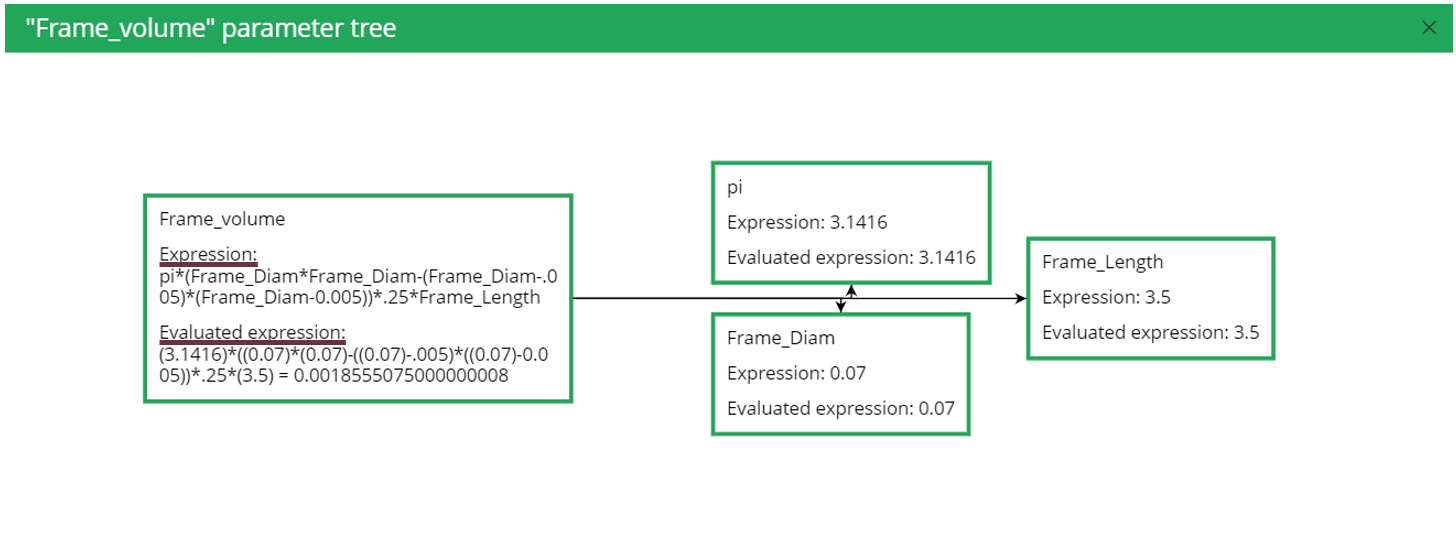<
Index# What is the parameter calculation tree in SimaPro Flow?

## Information

 When using parameters in SimaPro Flow there are several interesting features, the parameter calculation tree is one of them. The parameter calculation tree gives information about which other parameters are involved in the calculation of a certain parameter. The tree can give hints about where in the calculation something is wrong or it is an easy way to check that the correct inputs and equations are being used. You can find this feature in the parameter page of your Flow project.As can be seen in the image below, both the expression and the evaluated expression are shown.The Expression depicts how the parameters are being used in the calculationThe Evaluated expression shows how corresponding numbers are being used in the calculation and the calculated result. If, while modeling, you get a parameter with the value 'NaN', this means that something is going wrong in the calculation, possibly a parameter that divides by zero. In that case, the parameter calculation tree will show, by marking the parameter box red, which parameter calculation is giving the NaN error. By checking the parameter calculation tree you can easily identify what is going wrong and adjust the parameters.